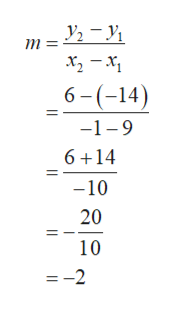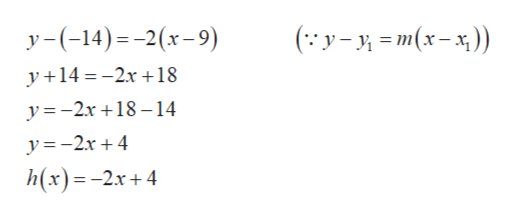find a linear functin h, given h(9)=-14 and h(-1)=6. Then find h(8). what's h(x)=.

Question

find a linear functin h, given h(9)=-14 and h(-1)=6. Then find h(8). what's h(x)=.

Step 1

The given points are, (9, –14) and (–1, 6).

Let y = h(x), (x1, y1) = (9, –14) and (x2 , y2) = (–1, 6).

The slope of the line is calculated as follows.help_outlineImage Transcriptionclosem 6-(-14) -1-9 6 14 -10 20 10 =-2 fullscreen
Step 2

Substitute the values x1 = 9, y1 = –14 and m = –2 in the line e...help_outlineImage Transcriptioncloseу -(-14)--2(х-9) (:У-и-т(х-х)) y 14=-2r18 y=2x +18 14 y=-2r4 h(x)%3D - 2х + 4 11 fullscreen

Want to see the full answer?

See Solution

Want to see this answer and more?

Our solutions are written by experts, many with advanced degrees, and available 24/7

See Solution
Tagged in

Equations and In-equations